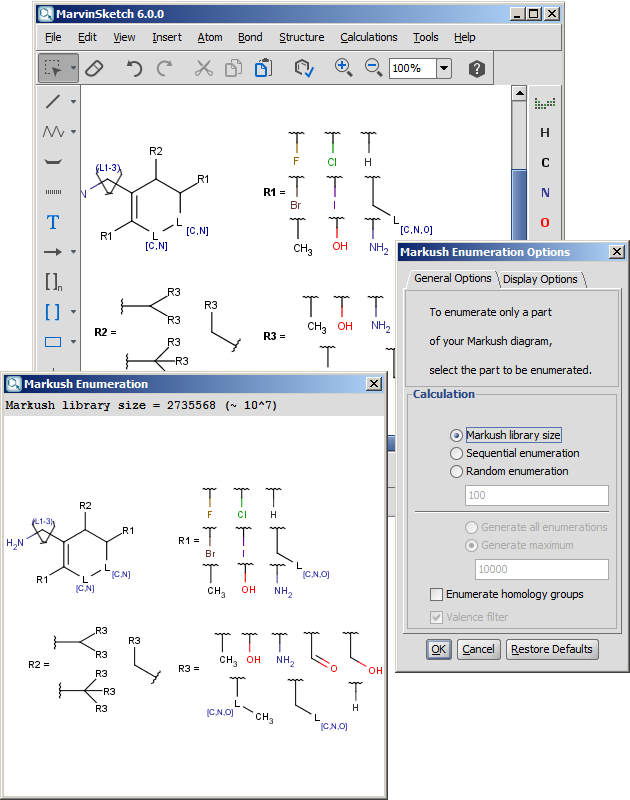# Calculate library size

The size of the Markush library can be calculated by arbitrary precision. On the user interface, the exact value is displayed until 20 digits, above that only the magnitude is shown (for example, 10^28). The calculated number is the size of the whole library, and does not consider the valence check filter. (See below.)

If the 'Enumerate homology groups' option is enabled, the number of enumerated molecules increases accordingly, multiplied by the number of built-in species.